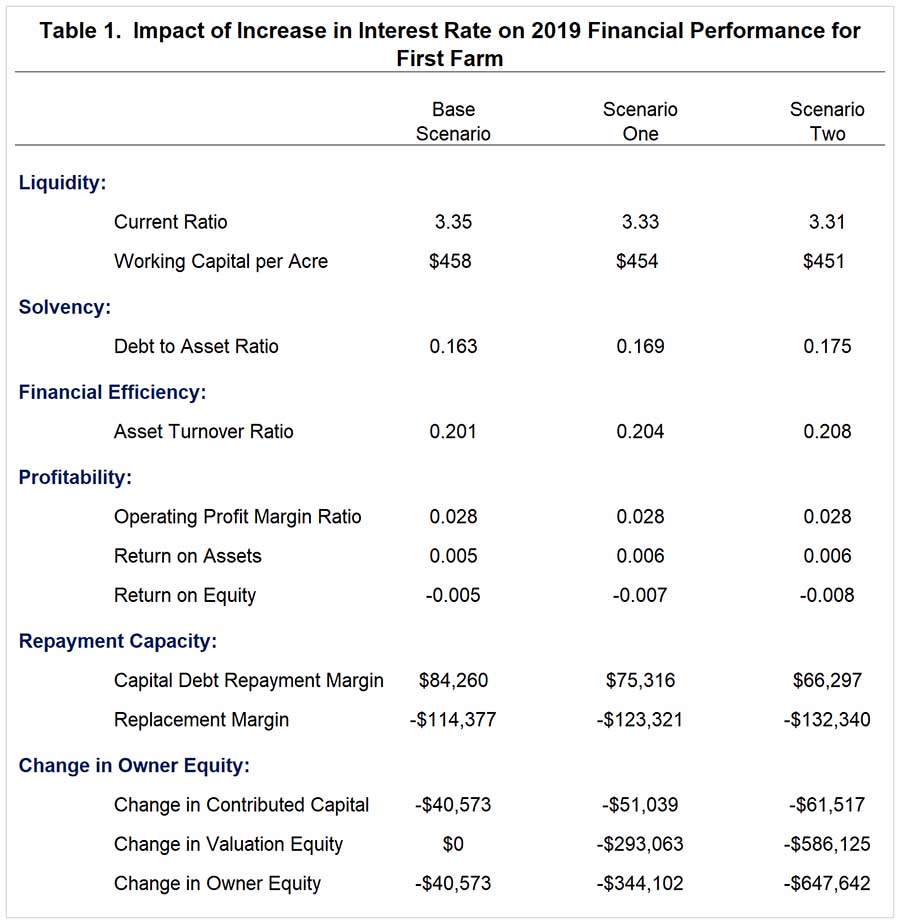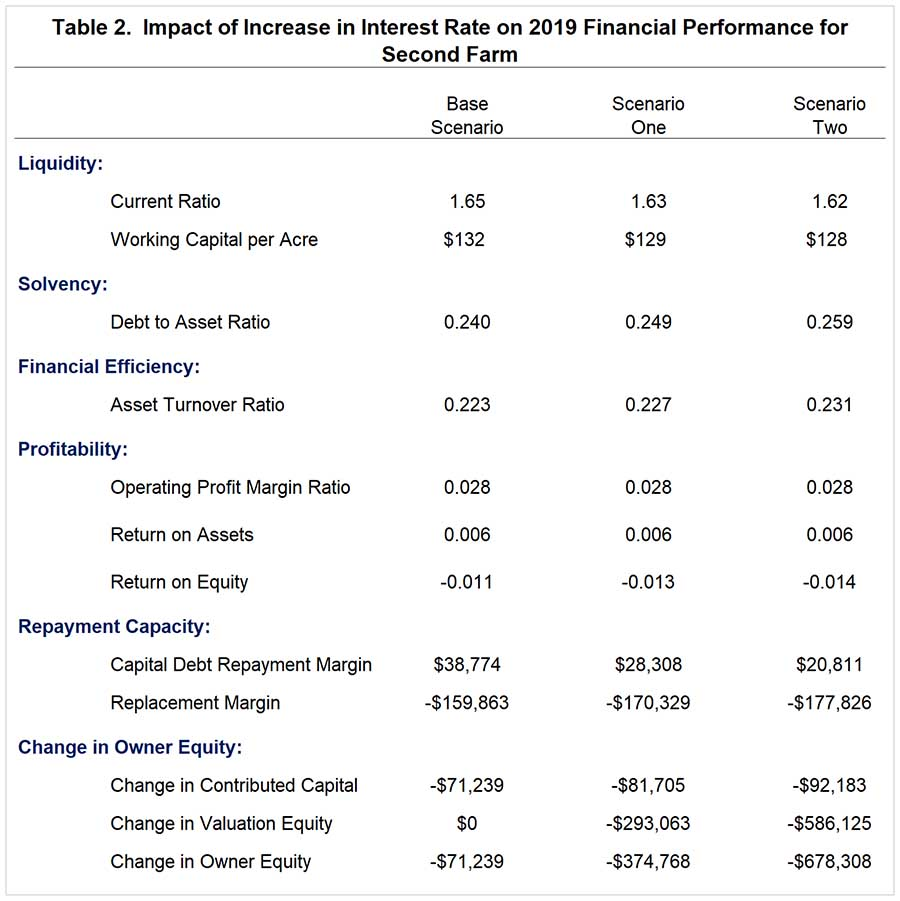# Using Stress Testing to Examine the Impact of an Increase in Interest Rates

November 2, 2018
farmdoc daily (8):203
Recommended citation format: Langemeier, M. "Using Stress Testing to Examine the Impact of an Increase in Interest Rates." farmdoc daily (8):203, Department of Agricultural and Consumer Economics, University of Illinois at Urbana-Champaign, November 2, 2018.

Stress testing describes a range of techniques that can be used to assess the vulnerability of a farm’s financial statements and performance to changes in prices, production, or financing.  Stress testing can be an extremely useful tool when evaluating strategies for dealing with lower prices, higher costs, asset purchases, and changes in loan terms.  In a previous article (farmdoc daily, March 4, 2016), we used a case farm in west central Indiana to examine the impact of a decline in gross revenue, and purchase of additional machinery on key financial ratios.  This article uses two case farms to examine the impact of an increase in interest rates on liquidity, solvency, financial efficiency, profitability, repayment capacity, and the change in owner equity.

#### Case Farm

Both case farms are located in west central Indiana, have 3000 acres, and utilize a corn/soybean rotation.  The case farms own 750 acres and cash rent the remaining acres.  The case farms participate in the ARC-CO program and purchase 80 percent revenue protection coverage.  The two case farms are assumed to have the same yields and cost structure.  The primary differences between the two farms involve their liquidity and solvency positions.

The first case farm has a very solid liquidity and solvency position.  Under the base scenario, involving no increase in interest rates, the projected current ratio and debt to asset ratio at the end of 2019 are 3.35 and 0.163, respectively.  The projected capital debt repayment capacity and replacement margin for 2019 are \$84,260 and -\$114,377.  The replacement margin is computed by subtracting cash used for capital replacement (depreciation multiplied by 1.15) from the capital debt repayment margin.  Projected depreciation expense and cash used for capital replacement in 2019 are \$172,728 and \$198,637, respectively.  The two repayment ratios indicate that the farm is covering principal and interest payments on term debt, but is not able to cover depreciation on machinery and equipment.  More information pertaining to repayment capacity ratios can be found in Langemeier (2018).

The second case farm has a much tighter liquidity position and has a higher debt to asset ratio.  Under the base scenario, involving no increase in interest rates, the projected current ratio and debt to asset ratio at the end of 2019 are 1.65 and 0.240, respectively.  The projected capital debt repayment capacity and replacement margin are \$38,774 and -\$159,043.  Though this farm can cover principal and interest payments, its cushion is approximately \$45,000 lower than that for the first case farm.

Two interest rate scenarios, an increase of 1 percent and an increase of 2 percent, are examined below.  The increases in interest rates impact interest on operating debt, interest on noncurrent notes payable (i.e., machinery loans), and land values.  Due to a fixed interest rate loan, the interest rate on the land note is not assumed to increase.  Based on computations of the author, each 1 percent increase in interest rates is assumed to reduce land values by 5 percent.  This represents the upper range of the impact of an increase in interest rates on land values.

#### Stress Testing Analysis

Abbreviations of the scenarios used for both case farms are as follows.  The base scenario assumes that interest rates in 2019 are the same as those experienced in 2018.  Scenario one assumes an increase of 1 percent in the interest rate for operating and machinery loans.  Scenario two assumes an increase of 2 percent in the interest rate for operating and machinery loans.

The results for the first case farm are presented in Table 1.  The impacts of the increases in interest rates on liquidity, solvency, financial efficiency, and profitability are relatively small.  The capital debt repayment margin and replacement margin are reduced by approximately \$8,900 with a 1 percent in interest rates and \$18,000 with a 2 percent increase in interest rates.  The cash used for capital replacement for this case farm is \$198,637 (depreciation multiplied by 1.15).  As illustrated in Table 1, the case farm can only cover a portion of capital replacement needs in 2019.  Not surprisingly, the change in equity is significantly impacted by increases in interest rates.  Owner equity declined 0.5 percent, 4.3 percent, and 8.2 percent under the base scenario, scenario one (1 percent increase in interest rates), and scenario two (2 percent increase in interest rate), respectively.Table 2 illustrates the impact of increasing interest rates by 1 percent and 2 percent for the second case farm.  As with the first case farm, the impacts of increases in interest rates on liquidity, solvency, financial efficiency, and profitability are relatively small.  For this case farm, the capital debt repayment margin and the replacement margin are reduced approximately \$10,500 and \$18,000 for the 1 percent and 2 percent interest rate increases, respectively.  Owner equity deterioration is even larger for the second case farm.  Owner equity declined 1.1 percent, 5.7 percent, and 10.4 percent under the base scenario, scenario one (1 percent increase in interest rates), and scenario two (2 percent increase in interest rates), respectively.After examining the results in tables 1 and 2, one may naturally ask why the impacts on liquidity and solvency were relatively small.  Let’s start by discussing the impacts on liquidity.  Given that interest rates are relatively low right now, it would take a larger increase in interest rates than 1 or 2 percent to impact net farm income significantly enough to create a large drop in liquidity.  With regard to solvency, land values in 2018 for the case farms are still more than double what they were in 2006.  Thus, it would take a larger drop in land values than 5 or 10 percent to create a large increase in the debt to asset ratio.